Case Based Questions (MCQ)

Chapter 8 Class 10 Introduction to Trignometry (Term 1)
Serial order wise

This question is inspired from Ex 9.1, 1 - Chapter 9 Class 10 - Applications of Trigonometry

## A circus artist is climbing from the ground along a rope stretched from the top of a vertical pole and tied at the ground. The height of the pole is 12 m and the angle made by the rope with ground level is 30°.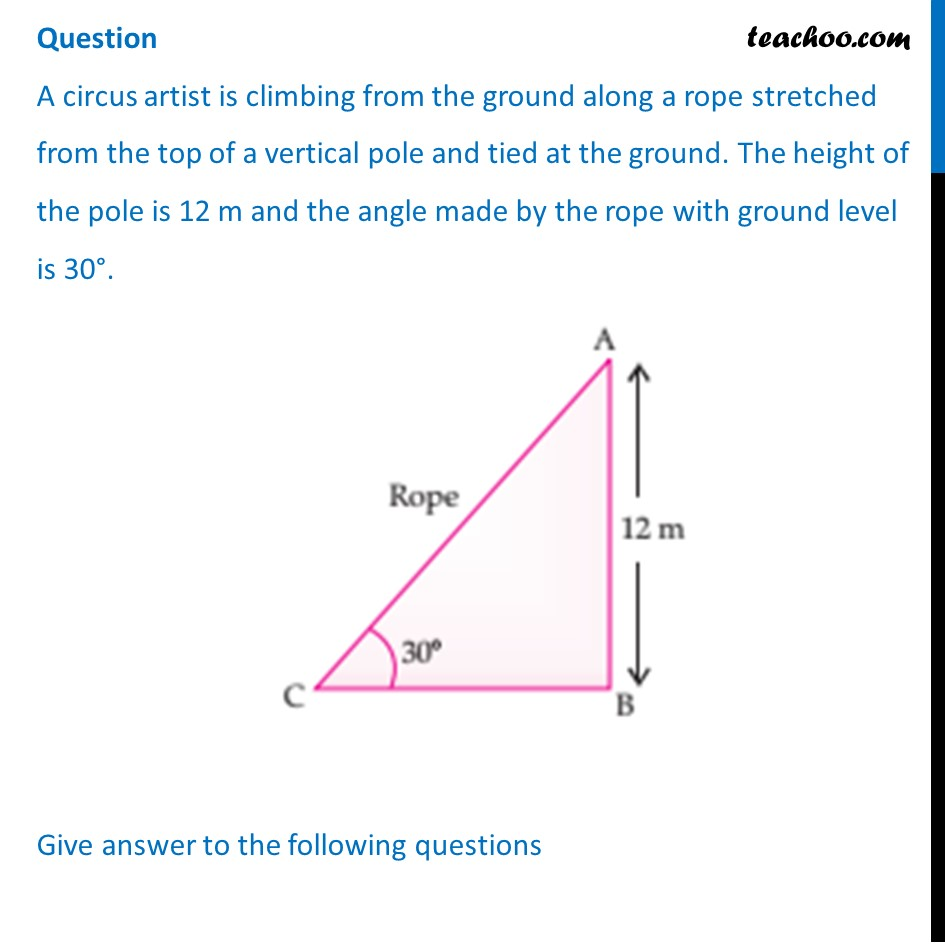## (c) 28 m                                                  (d) 22 m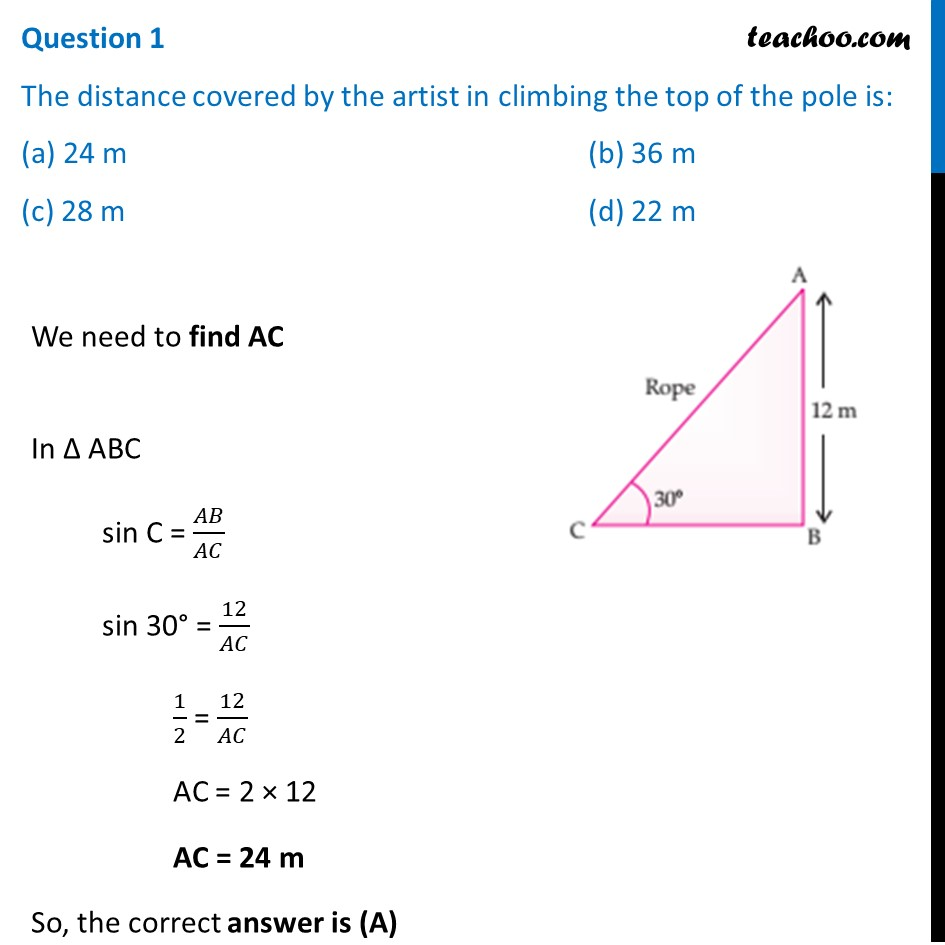## (c) 2√3 m     (d) √3 m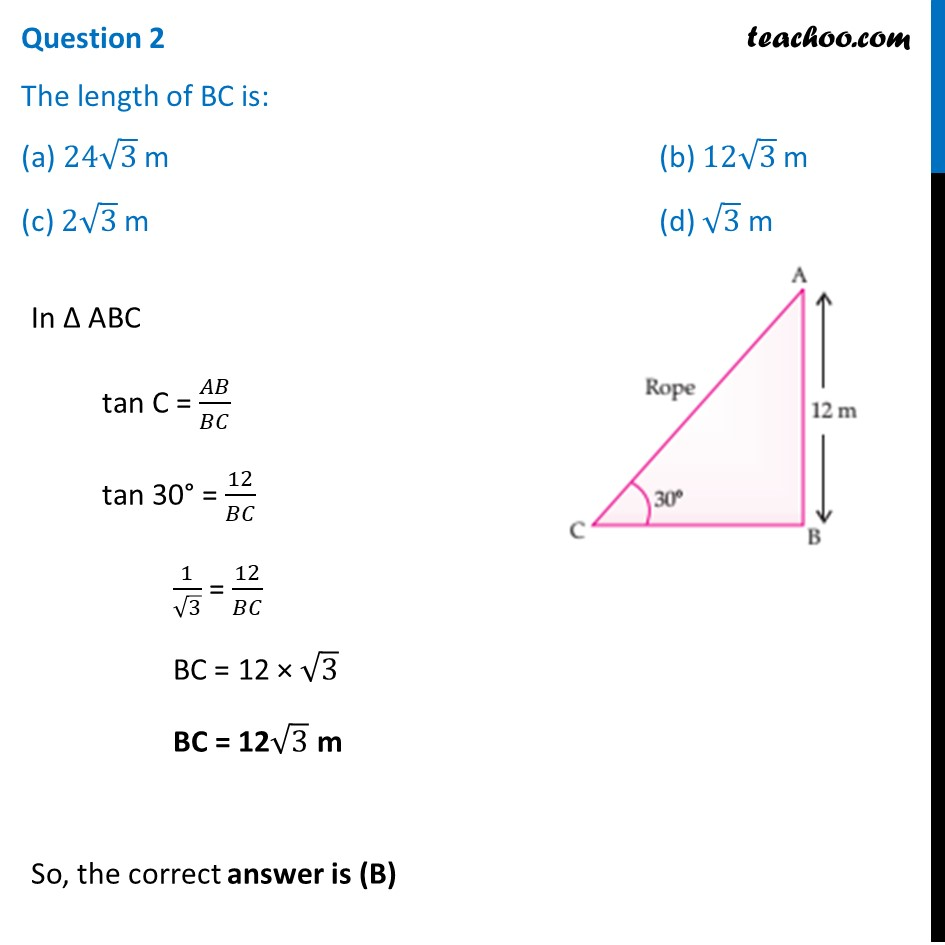## (c) 60°                                         (d) 45°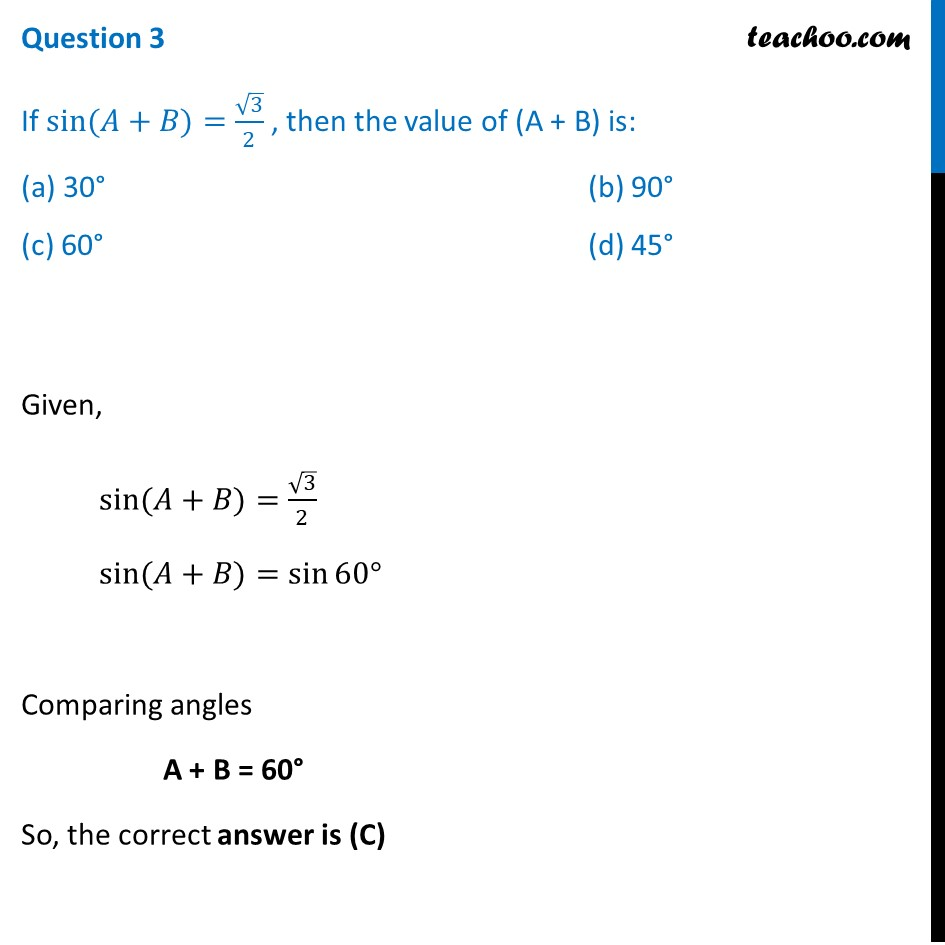## (c) 10                                                                 (d) 3/2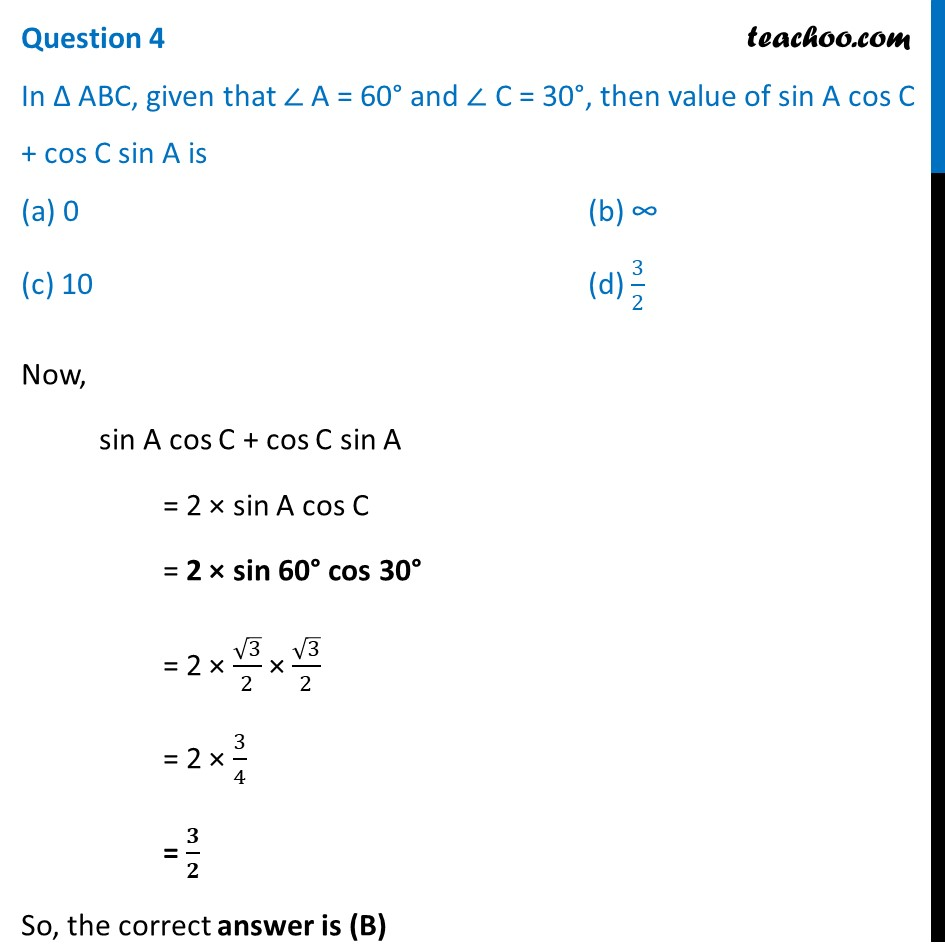## (c) Circle                                             (d) Mensuration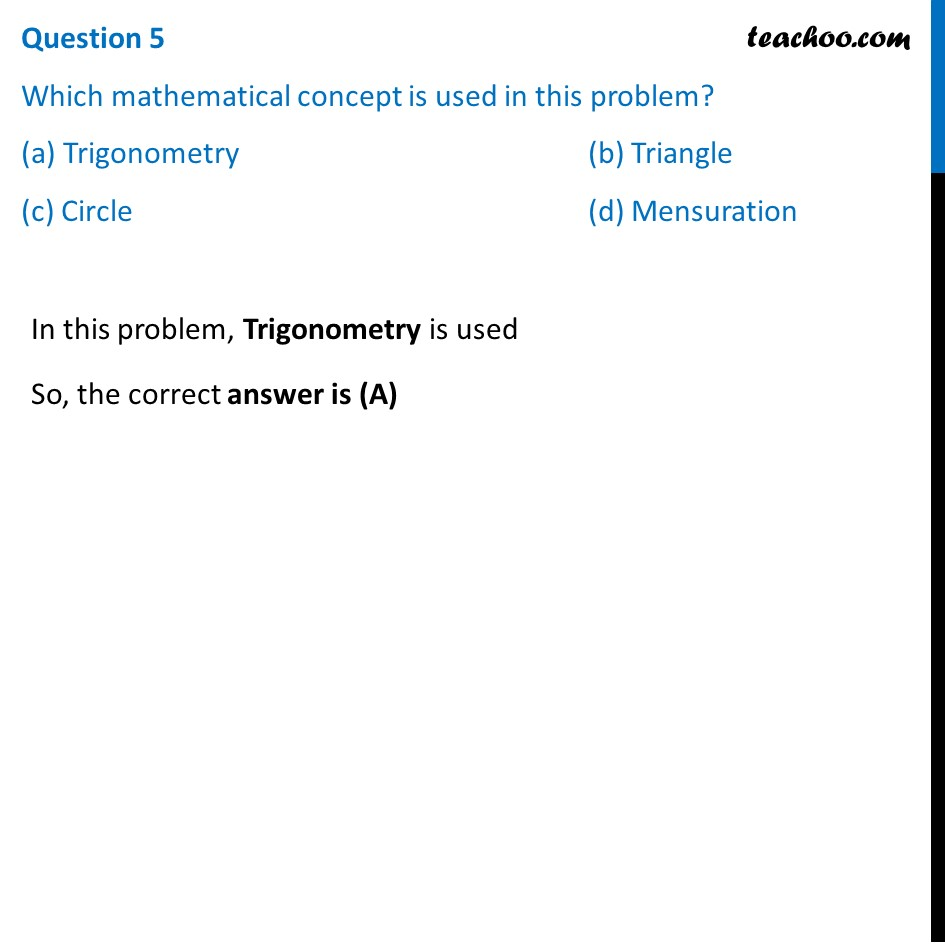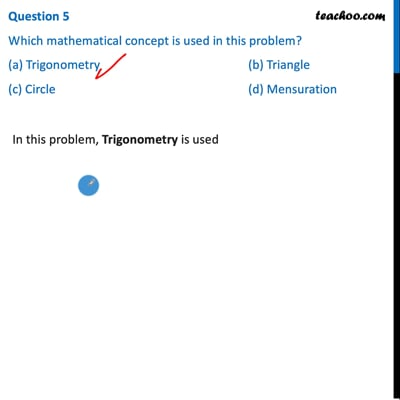This video is only available for Teachoo black users

### Transcript

Question A circus artist is climbing from the ground along a rope stretched from the top of a vertical pole and tied at the ground. The height of the pole is 12 m and the angle made by the rope with ground level is 30°. Give answer to the following questionsQuestion 1 The distance covered by the artist in climbing the top of the pole is: (a) 24 m (b) 36 m (c) 28 m (d) 22 m We need to find AC In Δ ABC sin C = 𝐴𝐵/𝐴𝐶 sin 30° = 12/𝐴𝐶 1/2 = 12/𝐴𝐶 AC = 2 × 12 AC = 24 m So, the correct answer is (A) Question 2 The length of BC is: (a) 24√3 m (b) 12√3 m (c) 2√3 m (d) √3 m In Δ ABC tan C = 𝐴𝐵/𝐵𝐶 tan 30° = 12/𝐵𝐶 1/√3 = 12/𝐵𝐶 BC = 12 × √3 BC = 12√3 m So, the correct answer is (B) Question 3 If sin⁡〖(𝐴+𝐵)〗=√3/2 , then the value of (A + B) is: (a) 30° (b) 90° (c) 60° (d) 45° Given, sin⁡〖(𝐴+𝐵)〗=√3/2 sin⁡〖(𝐴+𝐵)〗=sin⁡〖60° 〗 Comparing angles A + B = 60° So, the correct answer is (C) Question 4 In Δ ABC, given that ∠ A = 60° and ∠ C = 30°, then value of sin A cos C + cos C sin A is (a) 0 (b) ∞ (c) 10 (d) 3/2Now, sin A cos C + cos C sin A = 2 × sin A cos C = 2 × sin 60° cos 30° = 2 × √3/2 × √3/2 = 2 × 3/4 = 𝟑/𝟐 So, the correct answer is (B) Question 5 Which mathematical concept is used in this problem? (a) Trigonometry (b) Triangle (c) Circle (d) MensurationIn this problem, Trigonometry is used So, the correct answer is (A)